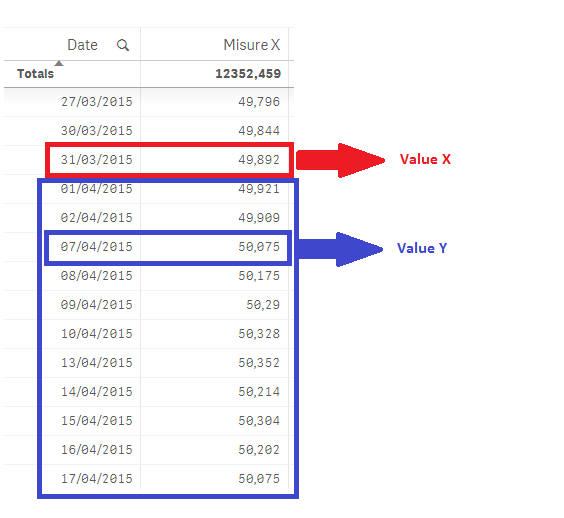# New to Qlik Sense

Discussion board where members can get started with Qlik Sense.

Announcements
Qlik® Product Spotlight: Discover what’s possible. Get more from our products.
See for yourself. Register today.Not applicable

## Set Analysis to calculate last day of previous month.

Hi all,

I show you a table with 2 columns: Date and one Misure.The column Date contains dates in this format "DD/MM/YYY" and the column "Misure X" the corrispondent value of each day.

I need to calculate this formula:

((Value Y - Value X) / Value X) * 100

as it is shown in the picture.

Value X is the value of the last day of the previous month of the month selected by the user.

Value Y is the value of each day of the selected month by the user.

I need to calculate the formula for each day of the selected month by the user, divi

For example:

- Value X = 49,892 (corrispondent value of the last day of the previous month of the month selected by the user)

01/04/2015 ---> ((49,921/49,892)/49,892) * 100

02/04/2015 ---> ((49,909/49,892)/49,892) * 100

07/04/2015 ---> ((50,075/49,892)/49,892) * 100

...etc...

I tried to calculate the last day of the previous month with this expression

```date(max(addmonths (mydate,-1,1)))
```

And it works.

I tried to calculate the corrispondent value of this date, but I have a problem to formulate a SetAnalysis expression to calculate the entire formula.

Can someone help me, please?

Thank you.

Tags (3)
2 Replies
Not applicable

## Re: Set Analysis to calculate last day of previous month.

I think the most ideal way to do this would be to use The As-Of Table

Not applicable

## Re: Set Analysis to calculate last day of previous month.

Ciao Giuseppe

simply use three variables:

1. vLastDayPreviousMonth=max( {<Mese={'\$(#vMonth)'}, Data=>} Data)

2. vMonth=max(Mese)-1   where Mese is num(month(Data)) as Mese

3. vValuePreviousMonth =sum({< Data ={'\$(#vLastDayMonth)'}, Mese=>} MisureX)

In the chart you will create an expression like  (sum(MisureX)/vValue)-1

You'll have to select only the month (Mese).

Ciao

Community Browser